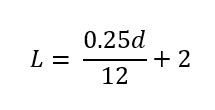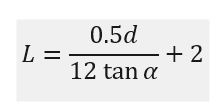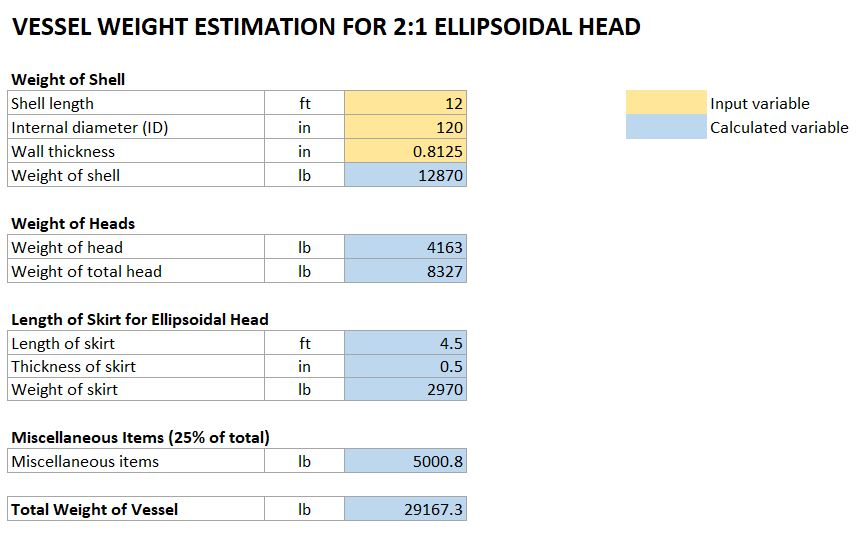# How To Estimate Vessel Weight

Estimating vessel weight is not usual daily task of a process engineer. However, I found it useful to know how to estimate vessel weight, when we need to estimate vessel cost. In other chance, civil engineer also asks about vessel weight to design the equipment foundation.

To better estimate vessel weight, we need to understand vessel weight components. Those are weight of the shell, the weight of the heads, and the weight of internals, nozzles, pedestals, and skirts.

The shell weight can be estimated by using the following equation:

W = 11 d t L

Where:

W           = weight, lb

d             = internal diameter (ID), in

t              = wall thickness, in

L             = shell length, ft

The weight of one 2:1 ellipsoidal head is approximately:

W ≈ 0.34 t d2 + 1.9 td

The weight of one cone is:

α            = one half cone apex angle

The weight of nozzles and internals can be estimated at 5% to 10% of the sum of the shell and head weights. The weight of a skirt can be estimated as the same weight per foot as the shell with a length given by equations below.Where L = skirt length, ft

The weight of pedestals for a horizontal vessel can be estimated as 10% of the total weight of the vessel.